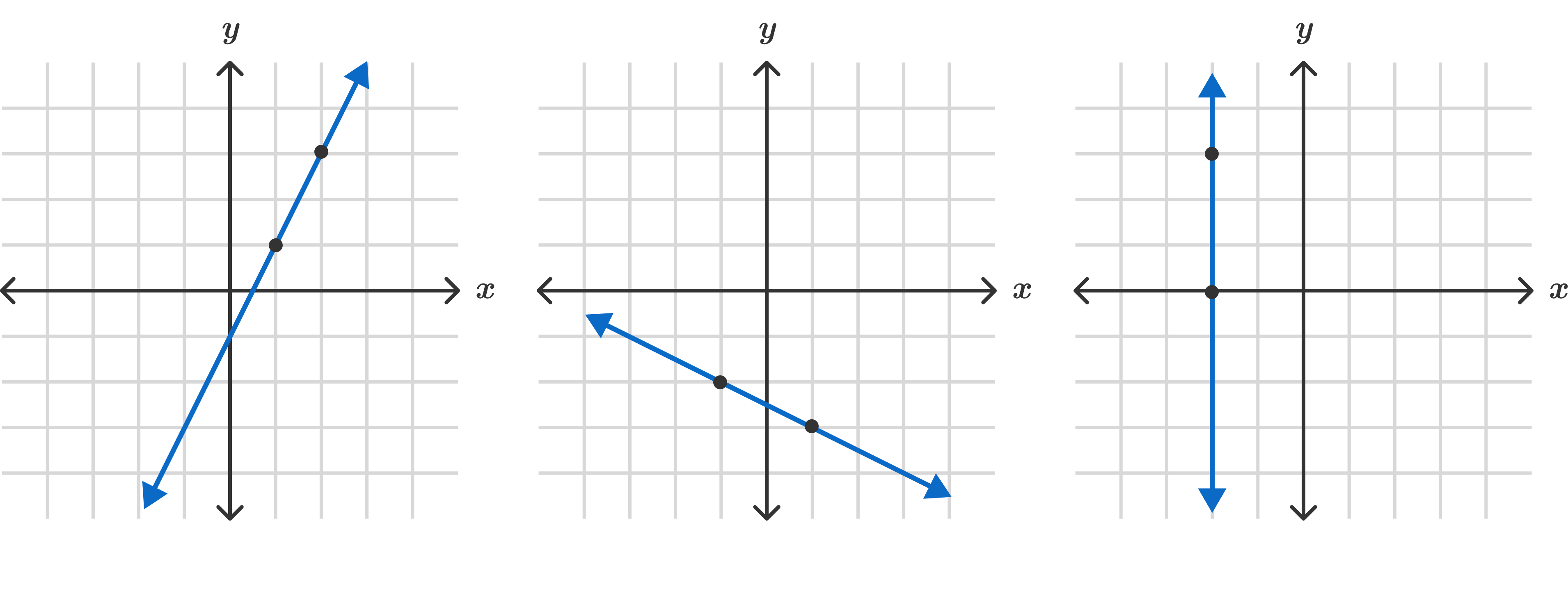Algebra I

The slider below modifies one of the parameters of a linear equation. What could we reasonably expect to happen to the line if the slider were to move to a negative value?

Line Exploration

These steepness (called the slope) of the two lines are controlled by the sliders below. What would happen to the lines if the sliders had the same value?

Hint: pay attention to the value labels on the sliders; even if the sliders are in the same position, they may not correspond to the same value.

Line Exploration

The slider below controls the slope of all the lines. If the lines below are described by equations in the form $y=mx+b$, which parameter is the slider controlling?

Line Exploration

In the equation $y=kx$, any change to the value of $x$ results in a change to the value of $y$.

If adding $3$ to $x$ results in $y$ growing by $12$, what is the value of $k$?

Line ExplorationThe equation that powers the visualization below is $y=mx + b$. $m$ controls the slope of the line and $b$ controls its position. Is it possible to make every line shown above in the format $y = mx + b ?$

Line Exploration

We want the line shown to pass through the marked red point at $(2,2) .$ Which values of $m$ will work? Mark all that apply and note that $b$ can vary. $y = mx + b$

Line Exploration

Select one or more

×